# CONFIDENCE INTERVALS

It is always important to have some idea of the size and influence of random errors. It is often important to be able to communicate this idea to others in the form of a statement such as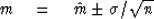In a matter of any controversy you may be called upon to define a probability that the true mean lies in your stated interval; in other words, what is your confidence that m lies in the interval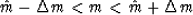Before you can answer questions about probability, it is necessary to make some assumptions and assertions about the probability functions which control your random errors. The assertion that errors are independent of one another is your most immediate hazard. If they are not, as is often the case, you may be able to readjust the numerical value of n to be an estimate of the number of independent errors. We did something like this in time series analysis when we took n to be not the number of points on the time series but the number of intervals of length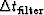.The second big hazard in trying to state a confidence interval is the common assumption that, because of the central-limit theorem and for lack of better information, the errors follow a gaussian probability function. If in fact the data errors include blunders which arise from human errors or blunders from transient electronic equipment difficulties, then the gaussian assumption can be very wrong and can lead you into serious errors in geophysical interpretation. Some useful help is found in the field of nonparametric statistics.

To begin with, it is helpful to rephrase the original question into one involving the median rather than the mean. The median m1 is defined as that value which is expected to be less than half of the population and greater than the other half. In many--if not most--applications the median is a ready, practical substitute for the arithmetic mean. The median is insensitive to a data point, which, by some blunder, is near infinity. In fact, median and mean are equal when the probability function is symmetrical. For a sample of n numbers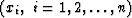,the median m1 may be estimated by reordering the numbers from smallest to largest and then selecting the number in the middle as the estimate of the median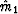.Specifically, let the recordered xi be denoted by xi' where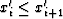.Then we have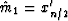.Now it turns out that without knowledge of the probability density function for the random variables xi we will still be able to compute the probability that the true median m1 is contained in the interval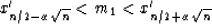(71)
For example, set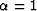and N = 100, the assertion is that we can now calculate the probability that the true median m1 lies between the 40th and the 60th percentile of our data. The trick is this:  Define a new random variable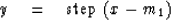(72)
The step function equals +1 if x > m1 and equals if x < m1. The new random variable y takes on only values of zero and one with equal probability; thus we know its probability function even though we may not know the probability function for the random variable x. Now define a third random variable s as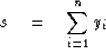Since each yi is zero or one, then s must be an integer between zero and n. Further more, the probability that s takes the value j is given by the coefficient of Zj of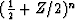. Now the probability that s lies in the interval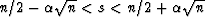is readily determined by adding the required coefficients of Zj, and this probability is by definition equal to the probability that the median m1 lies in the interval (71). Forand large n this probability works out to about 95 percent.